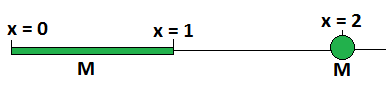# Gravitationally Equivalent Point MassThere are two objects, a thin rod of mass $M$ in the interval $[0,1]$ on the $x$-axis, and a point mass at $x=2$ of the same mass $M$, as shown above.

Suppose we wanted to replace the rod with a particle of mass $M$, which would exert the same gravitational force on the point particle at $x = 2$.

What should its approximate $x$-coordinate be?

×